# Depth of a module

(diff) ← Older revision | Latest revision (diff) | Newer revision → (diff)

One of the cohomological characteristics of a module over a commutative ring. Letbe a Noetherian ring, letbe an ideal in it and letbe an-module of finite type. Then the-depth of the moduleis the least integerfor which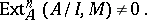The depth of a module is denoted byor by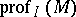. A different definition can be given in terms of an-regular sequence, i.e. a sequence of elementsofsuch that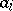is not a zero divisor in the moduleThe-depth ofis equal to the length of the largest-regular sequence consisting of elements of. The maximal ideal is usually taken forin the case of a local ring. The following formula is valid: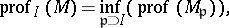where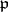denotes a prime ideal in, while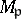is considered as a module over the local ring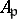.

The concept of the depth of a module was introduced in  under the name of homological codimension. If the projective dimensionof a moduleover a local ringis finite, then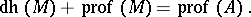In generalis not larger than the dimension of.

The depth of a module is one of the basic tools in the study of modules. Thus, Cohen–Macaulay modules and rings (cf. Cohen–Macaulay ring) have been defined in terms of the depth of modules. The Serre criterion () for an-module: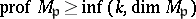for all prime idealsin, often proves to be useful. Finally, the depth of modules is closely connected with local cohomology modules: The statementis equivalent to saying that the local cohomology modules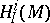vanish if.

How to Cite This Entry:
Depth of a module. Encyclopedia of Mathematics. URL: http://encyclopediaofmath.org/index.php?title=Depth_of_a_module&oldid=17353
This article was adapted from an original article by V.I. Danilov (originator), which appeared in Encyclopedia of Mathematics - ISBN 1402006098. See original article